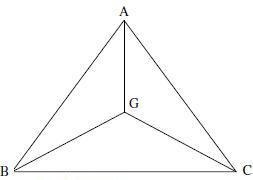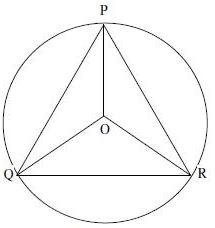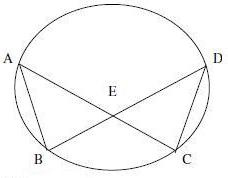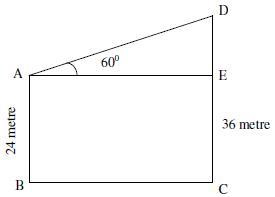# Sample Aptitude Questions of SAMSUNG

1. If G is the centroid of △ABC and △ABC = 48 cm2, then the area of △BGC is
1. 16 cm2
2. 24 cm2
3. 31 cm2
4. 8 cm2△BGC = 1/3 * △ABC = 1/3 * 48 = 16 sq. cm
2. The diagonals AC and BD of a cyclic quadrilateral ABCD intersect each other at the point P. Then, it is always true that
1. AP x BP = CP x DP
2. AP x CP = AB x CP
3. BP x AB = CD x CP
4. AP x CP = BP x DPHere, AC and BD are chords of the circle so AP x PC = BP x PD.
1. If tan Θ = 3/4 and Θ is acute, then cosec Θ is
1. 5/4
2. 4/3
3. 4/5
4. 5/3

tan Θ=3/4
cot Θ = 4/3
cosec2Θ - cot2Θ = 1
cosec Θ= √ 1+cot2Θ = √ 1+(4/3)2 = √1+16/9=√25/9=5/3
2. The value of is: 1/(1+tan2)Θ)+1/(1+cot2
1. 2
2. 1/2
3. 1/4
4. 1

Expression
=1/1+tan2Θ+1/1+cot2Θ
1/1+sec2Θ+1/1+cosec2Θ
Cos2Θ+ sin2Θ = 1
3. If O be the circum centre of a triangle PQR and ∠QOR = 110 °, ∠OPR = 25 °, then the measure of ∠PRQ is
1. 55 °
2. 60 °
3. 65 °
4. 50 °∠QOR = 110 ° ∠OPR = 250 ° ∠QPR = 110 ° / 2 = 55 ° OR = OP ∠OPR = ∠PRO = 25° ∠OQR = ∠ORQ = 70/2 = 35 ° PRQ = 25 ° + 35 ° = 60 °
4. A vertical stick 12 cm long casts a shadow 8 cm long on the ground. At the same time, a tower casts a shadow 40m long on the ground. The height of the tower is
1. 65m
2. 70m
3. 72m
4. 60m

height of Tower/Length Of Stick
h/12=40/8
h=40*12/8 = 60 metre
5. A, B, C, D are four points on a circle. AC and BD intersect at a point E such that ∠BEC = 1300 and ∠ECD = 200. ∠BAC is
1. 100 °
2. 110 °
3. 120 °
4. 90 °∠BEC = 130 °
∠DEC = 180 ° - 130 ° = 50 °
∠EDC = 180 °-50 ° -20 ° = 110 °
∠BAC = ∠EDC = 110 °
(Angle on the same arc)
6. In a triangle, if three altitudes are equal, then the triangle is
1. Right
2. Isosceles
3. Obtuse
4. Equilateral

The only possible case is when the Triangle is equilateral.
7. The tops of two poles of height 24m and 36 m are connected by a wire. If the wire makes an angle of 60 ° with the horizontal, then the length of the wire is
1. 8 m
2. 6 √3 m
3. 6 m
4. 8√3mDE = 36-24 = 12 metre